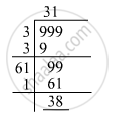Advertisement Remove all ads

# Find the Greatest Number of Three Digits Which is a Perfect Square. - Mathematics

Find the greatest number of three digits which is a perfect square.

Advertisement Remove all ads

#### Solution

The greatest number with three digits is 999. To find the greatest perfect square with three digits, we must find the smallest number that must be subtracted from 999 in order to get a perfect square. For that, we have to find the square root by the long division method as shown below:So, 38 must be subtracted from 999 to get a perfect square.
999 − 38 = 961
961 = 312
Hence, the greatest perfect square with three digits is 961.

Is there an error in this question or solution?
Advertisement Remove all ads

#### APPEARS IN

RD Sharma Class 8 Maths
Chapter 3 Squares and Square Roots
Exercise 3.5 | Q 11 | Page 44
Advertisement Remove all ads

#### Video TutorialsVIEW ALL 

Advertisement Remove all ads
Share
Notifications

View all notifications

Forgot password?
Course